Traversal means visiting all the nodes of a graph. Breadth first traversal or Breadth first Search is a recursive algorithm for searching all the vertices of a graph or tree data structure. In this article, you will learn with the help of examples the BFS algorithm, BFS pseudocode and the code of the breadth first search algorithm with implementation in C++, C, Java and Python programs.

## BFS algorithm

A standard DFS implementation puts each vertex of the graph into one of two categories:

1. Visited
2. Not Visited

The purpose of the algorithm is to mark each vertex as visited while avoiding cycles.

The algorithm works as follows:

1. Start by putting any one of the graph's vertices at the back of a queue.
2. Take the front item of the queue and add it to the visited list.
3. Create a list of that vertex's adjacent nodes. Add the ones which aren't in the visited list to the back of the queue.
4. Keep repeating steps 2 and 3 until the queue is empty.

The graph might have two different disconnected parts so to make sure that we cover every vertex, we can also run the BFS algorithm on every node

## BFS example

Let's see how the Breadth First Search algorithm works with an example. We use an undirected graph with 5 vertices.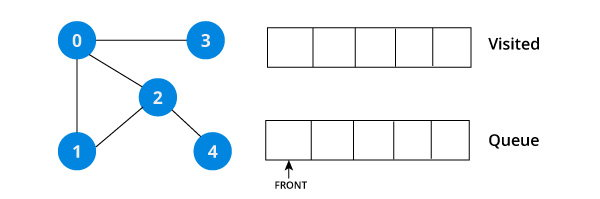We start from vertex 0, the BFS algorithm starts by putting it in the Visited list and putting all its adjacent vertices in the stack.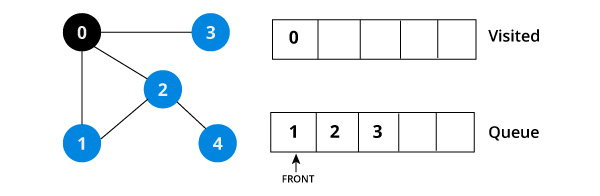Next, we visit the element at the front of queue i.e. 1 and go to its adjacent nodes. Since 0 has already been visited, we visit 2 instead.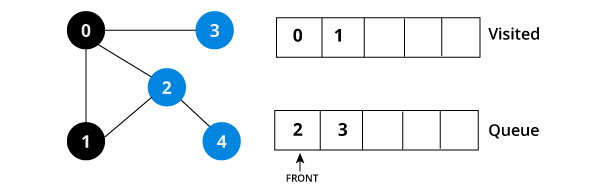Vertex 2 has an unvisited adjacent vertex in 4, so we add that to the back of the queue and visit 3, which is at the front of the queue.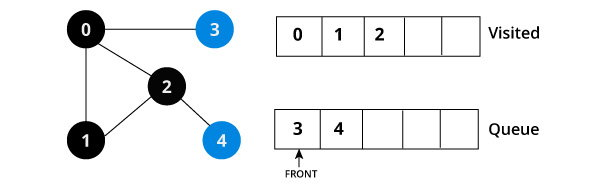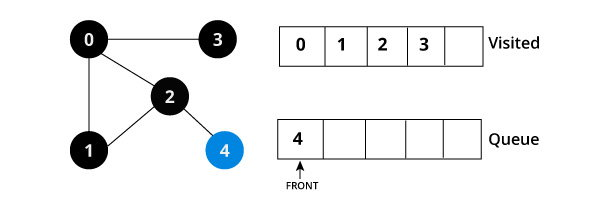Only 4 remains in the queue since the only adjacent node of 3 i.e. 0 is already visited. We visit it.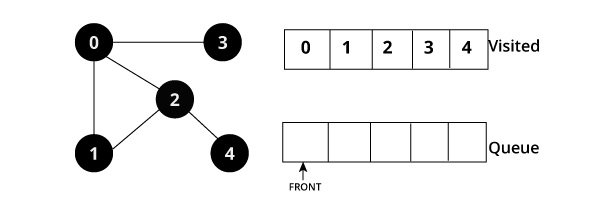Since the queue is empty, we have completed the Depth First Traversal of the graph.

## BFS pseudocode

```create a queue Q
mark v as visited and put v into Q
while Q is non-empty
remove the head u of Q
mark and enqueue all (unvisited) neighbours of u```

## BFS code

The code for the Breadth First Search Algorithm with an example is shown below. The code has been simplified so that we can focus on the algorithm rather than other details.

### BFS in C

``````#include <stdio.h>
#include <stdlib.h>
#define SIZE 40

struct queue {
int items[SIZE];
int front;
int rear;
};

struct queue* createQueue();
void enqueue(struct queue* q, int);
int dequeue(struct queue* q);
void display(struct queue* q);
int isEmpty(struct queue* q);
void printQueue(struct queue* q);

struct node
{
int vertex;
struct node* next;
};

struct node* createNode(int);

struct Graph
{
int numVertices;
int* visited;
};

struct Graph* createGraph(int vertices);
void addEdge(struct Graph* graph, int src, int dest);
void printGraph(struct Graph* graph);
void bfs(struct Graph* graph, int startVertex);

int main()
{
struct Graph* graph = createGraph(6);

bfs(graph, 0);

return 0;
}

void bfs(struct Graph* graph, int startVertex) {

struct queue* q = createQueue();

graph->visited[startVertex] = 1;
enqueue(q, startVertex);

while(!isEmpty(q)){
printQueue(q);
int currentVertex = dequeue(q);
printf("Visited %d\n", currentVertex);

while(temp) {

}
temp = temp->next;
}
}
}

struct node* createNode(int v)
{
struct node* newNode = malloc(sizeof(struct node));
newNode->vertex = v;
newNode->next = NULL;
return newNode;
}

struct Graph* createGraph(int vertices)
{
struct Graph* graph = malloc(sizeof(struct Graph));
graph->numVertices = vertices;

graph->adjLists = malloc(vertices * sizeof(struct node*));
graph->visited = malloc(vertices * sizeof(int));

int i;
for (i = 0; i < vertices; i++) {
graph->visited[i] = 0;
}

return graph;
}

void addEdge(struct Graph* graph, int src, int dest)
{
// Add edge from src to dest
struct node* newNode = createNode(dest);

// Add edge from dest to src
newNode = createNode(src);
}

struct queue* createQueue() {
struct queue* q = malloc(sizeof(struct queue));
q->front = -1;
q->rear = -1;
return q;
}

int isEmpty(struct queue* q) {
if(q->rear == -1)
return 1;
else
return 0;
}

void enqueue(struct queue* q, int value){
if(q->rear == SIZE-1)
printf("\nQueue is Full!!");
else {
if(q->front == -1)
q->front = 0;
q->rear++;
q->items[q->rear] = value;
}
}

int dequeue(struct queue* q){
int item;
if(isEmpty(q)){
printf("Queue is empty");
item = -1;
}
else{
item = q->items[q->front];
q->front++;
if(q->front > q->rear){
printf("Resetting queue");
q->front = q->rear = -1;
}
}
return item;
}

void printQueue(struct queue *q) {
int i = q->front;

if(isEmpty(q)) {
printf("Queue is empty");
} else {
printf("\nQueue contains \n");
for(i = q->front; i < q->rear + 1; i++) {
printf("%d ", q->items[i]);
}
}
}``````

## Breadth First Search in C++

``````#include <iostream>
#include <list>

using namespace std;

class Graph
{
int numVertices;
bool* visited;
public:
Graph(int vertices);
void BFS(int startVertex);
};

Graph::Graph(int vertices)
{
numVertices = vertices;
}

{
}

void Graph::BFS(int startVertex)
{
visited = new bool[numVertices];
for(int i = 0; i < numVertices; i++)
visited[i] = false;

list queue;

visited[startVertex] = true;
queue.push_back(startVertex);

list::iterator i;

while(!queue.empty())
{
int currVertex = queue.front();
cout << "Visited " << currVertex << " ";
queue.pop_front();

{
{

}
}
}
}

int main()
{
Graph g(4);

g.BFS(2);

return 0;
}``````

### BFS Java code

``````import java.io.*;
import java.util.*;

class Graph
{
private int numVertices;
private boolean visited[];

Graph(int v)
{
numVertices = v;
visited = new boolean[numVertices];
for (int i=0; i i = adjLists[currVertex].listIterator();
while (i.hasNext())
{
{
}
}
}
}

public static void main(String args[])
{
Graph g = new Graph(4);

System.out.println("Following is Breadth First Traversal "+
"(starting from vertex 2)");

g.BFS(2);
}
}``````

## BFS in Python

``````import collections

def bfs(graph, root):
visited, queue = set(), collections.deque([root])